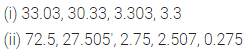# ML Aggarwal Class 6 Solutions for ICSE Maths Chapter 7 Decimals Ex 7.2

## ML Aggarwal Class 6 Solutions for ICSE Maths Chapter 7 Decimals Ex 7.2

Question 1.
Write the following decimal fractions as decimal numbers: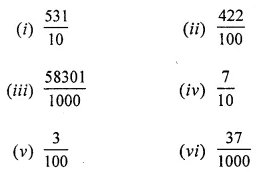Solution:Question 2.
Write the following decimal numbers as decimal fractions:
(i) 54.01
(ii) 318.105
(iii) 0.37
(iv) 0.047
(v) 0.03
(vi) 34.5
Solution: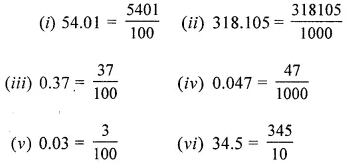Question 3.
Write the following decimal numbers as fractions in lowest terms:
(i) 0.8
(ii) 0.04
(iii) 0.125
(iv) 0.225
(vi) 0.066
(vii) 0.092
Solution:Question 4.
Convert the following decimal numbers into mixed fractions:
(i) 31.6
(ii) 3.25
(iii) 7.025
(iv) 95.95
Solution:Question 5.
Convert the following fractions into decimal numbers:Solution: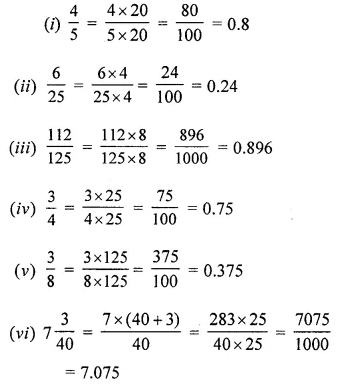Question 6.
Convert the following unlike decimal numbers to like decimal numbers :
(i) 17.5, 3.912
(ii) 5.04, 13.1902
(iii) 2.451, 3.7, 28.34
(iv) 3.1,2.678,27.0103
Solution: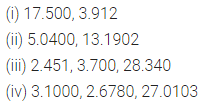Question 7.
In each of the following pairs of decimal numbers, state which number is greater ?
(i) 0.3, 0.4
(ii) 1, 0.99
(iii) 1.09, 1.093
(iv) 0.5, 0.05
Solution:Question 8.
In each of the following pairs of decimal numbers, state which number is smaller:
(i) 45.78, 345.8
(ii) 37.701, 37.71
(iii) 5.907, 5.903
Solution:Question 9.
Arrange the following decimal numbers in ascending order:
(i) 27.35, 27.305, 2.7, 2.543
(ii) 4.53, 4.07, 29.1, 0.9, 0.709
Solution: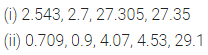Question 10.
Arrange the following decimal numbers in descending order:
(i) 3.303, 33.03, 3.3, 30.33
(ii) 72.5, 2.75, 27.505, 0.275, 2.507
Solution: